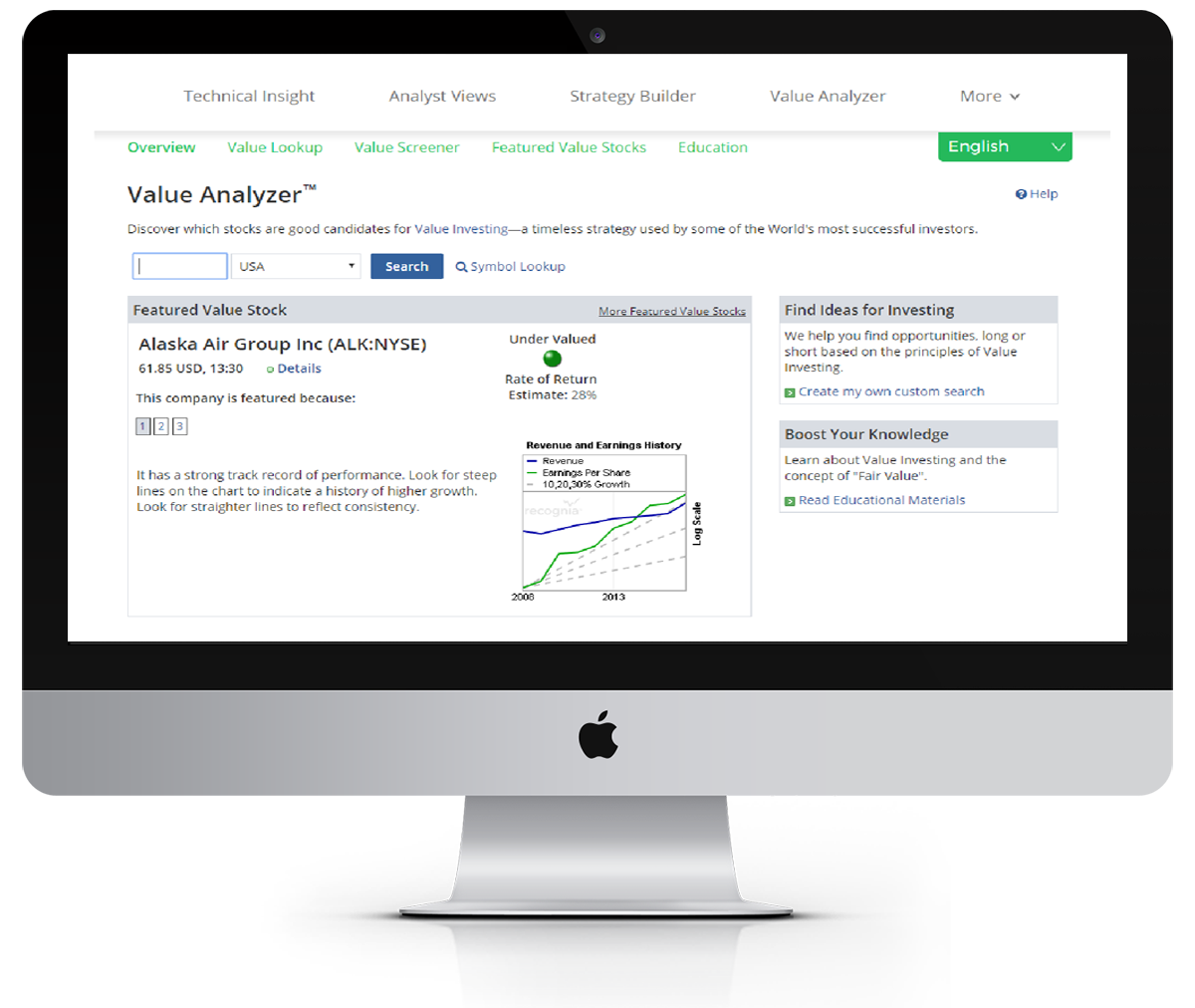Using Value Analyzer to understand
with
de
What factors are considered when calculating the fair value? Learn how Value Analyzer helps you understand a company's strength and fair value.
3
min
Quiz

## An essential concept in investing is that of Fair Value.

Fair Value is the appropriate price for the shares of a company, based on its earnings and growth rate. Fair Value is a theoretical calculation, that gives investors a starting point to work from when deciding how much to pay for a companies shares.

The most widely accepted calculation of Fair Value uses the idea that at Fair Value, the P/E ratio of a stock (Price/EPS), equals its Growth rate (EPS Growth)

The P/E ratio of any company that's fairly priced with equal its growth rate.

### Value Analyzer uses the Value Graph to show how a stock is priced relative to its Fair Value, both in the present and historically.

In order to make more realistic comparisons, Value Analyzer limits the estimated growth rate within a range of 0-40%. You may simulate different growth rates using the projector tool.

### The stock price is higher than the fair value.

If the stock price (indicated in purple) is over the fair value (shown by the green line) the stock is relatively expensive.

### The stock price is lower than the fair value.

If the stock price (indicated in purple) is under the fair value (shown by the green line) the stock is relatively inexpensive.

## Fair Value is an excellent tool, but it's not an exact science.

The calculation of Fair Value depends on the EPS growth rate of the company. This number is estimated as best as possible by Value Analyzer using historical data, but has built-in limitations:

1. The EPS growth rate number is estimated using several years of historical data.
2. Historical performance is not necessarily indicative of future performance.

There are other factors that can influence the valuation of a company. These include cash holdings, debt, property assets or patents etc.

After establishing the Fair Value calculation, it is important to follow up to make sure it is accurate, and understand what other factors will impact it.